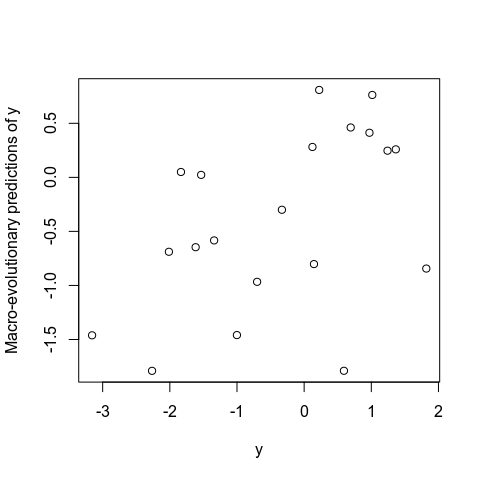# Analyzing rates of evolution

#### 2021-12-08

This vignette shows how to analyze rates of evolution using the comparative methods implemented in evolvability::rate_gls. The function rate_gls can fit three different types of evolutionary models depending on its model argument. In all three models the evolutionary rate of change in the y-variable is a function of the state of the predictor x. These models can be used to test hypotheses about the effect of an evolving trait (x) on the rate of evolution in a different trait (y). The different evolutionary models outlined below are described in detail in Hansen TF, Bolstad GH, Tsuboi M. 2021. Analyzing disparity and rates of morphological evolution with model-based phylogenetic comparative methods. Systematic Biology. syab079.

Note that sample sizes and number of simulations below are kept small in the interest of computational speed. With small sample sizes the parameter estimates will be inaccurate, and when performing a bootstrap in a real analysis the number of simulations is typically 1000. Hence, the below examples are only for explaining the functionality in the evolvability package.

To simulate data for the different evolutionary models, we first need an ultrametric phylogeny. Here we generate a random phylogeny using the ape package. In a real data analysis, you would use a molecular phylogeny of the species in your data set.

set.seed(999)
tree <- ape::rtree(n = 20)
tree <- ape::chronopl(tree, lambda = 1, age.min = 2)

Checking whether the phylogeny is ultrametric:

ape::is.ultrametric(tree)
#>  TRUE

We scale the phylogeny to unit length between root and tips. This makes the parameters easier to interpret. (In our simulation this could also have been accomplished by setting age.min = 1 in chronopl).

tree$edge.length <- tree$edge.length/diag(ape::vcv(tree))
plot(tree)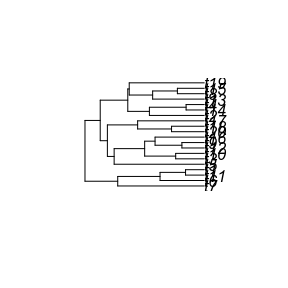# Predictor as Brownian motion

The first model is a model where the predictor $$x$$ evolves according to a Brownian motion (BM) process, while $$y$$ follows a Brownian motion with variance that is linear in $$x$$. This is referred to as model 1 in Hansen et al. (2021). The evolutionary model is

$dy = \sqrt{a + bx} dW_1$

$dx = \sigma dW_2$

where $$a$$ is the evolutionary rate of $$y$$ at $$x=0$$, $$b$$ captures the influence of $$x$$ on the evolutionary rate, and the $$dW_i$$ are two uncorrelated white noise processes. This model will break down when $$a + bx$$ becomes negative and should be seen as an approximation that may be valid for a given range of $$x$$ values as spanned by the species to be used in the analysis.

Using the function simulate_rate with model = "predictor_BM" we can simulate data at the tips of the phylogeny according to this model:

sim_data <- simulate_rate(tree, startv_x=0, sigma_x=0.25, a=1, b=1, model = "predictor_BM")

In our simulation we have chosen the starting value (root value) of x as $$0$$ (startv_x=0) and the parameter $$a$$ is set to $$1$$ (a=1). This means that, the evolutionary rate of $$y$$ at the root is $$1$$. The $$b$$ parameter is also set to $$1$$ in the simulation (b=1). Hence, points along the phylogeny where $$x$$ evolves to take the value $$0.5$$, the rate for $$y$$ is given by $$\sqrt{a + bx} = \sqrt{1 + 0.5} = 1.22$$, while points along the phylogeny where $$x$$ evolves to take the value $$-0.5$$, the rate of $$y$$ is given by $$\sqrt{1 - 0.5} = 0.71$$. For $$x$$, the rate of evolution, $$\sigma$$, is constant across the whole phylogeny, and set to sigma_x=0.25 in the simulations.

The simulation gives a warning message if $$a + bx$$ becomes negative in parts of the tree. In these instances, the rate parameter of $$y$$, $$\sqrt{a + bx}$$, is assigned the value $$0$$ in the simulation. As long as the number of these instances is low, this should not pose a problem. We can plot the evolution of the rate of $$y$$ (i.e. $$\sqrt{a + bx}$$), as well as the values of $$y$$ and $$x$$ using the inbuilt plot.simulate_rate function:

par(mfrow = c(1,3))
plot(sim_data) # defaults to response = "rate_y"
plot(sim_data, response = "y")
plot(sim_data, response = "x")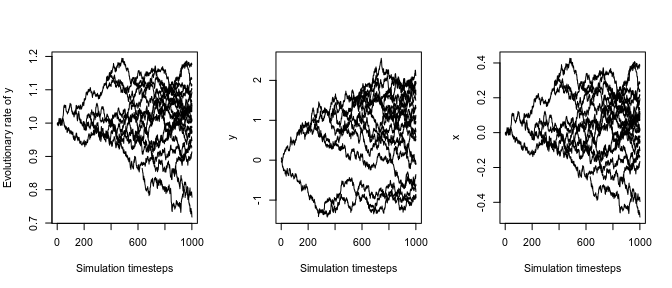As shown in the above figure, the simulation is discrete and done over 1000 time steps. The $$y$$ and $$x$$ values at the tips are stored as a data frame in sim_data$tips. d <- sim_data$tips
#>   species           x          y
#> 1      t7 -0.16512223 -0.3601518
#> 2      t6 -0.39554043 -0.9454565
#> 3     t11 -0.10247222 -0.9277121
#> 4      t1 -0.48436228 -0.6337186
#> 5      t5  0.02128758 -0.7865491
#> 6      t3  0.11798098  1.1257821

We can now fit the GLS (generalized least squares) model to these data and their phylogeny:

gls_mod <- rate_gls(x=d$x, y=d$y, species=d$species, tree, model = "predictor_BM", silent = TRUE) round(gls_mod$param, 3)
#>            Estimate    SE
#> a             1.240 0.336
#> b             2.259 2.543
#> sigma(x)^2    0.060 0.018

This output gives the GLS estimates of the parameters of the evolutionary model (sigma(x)^2 is the squared $$\sigma$$). Because of the low sample size, the estimates of the parameters diverges quite a lot from their true values (the input of the simulation). Note that the analytic standard error of $$a$$ and $$b$$ (shown in the SE column) does not take into account the error in $$\sigma^2$$. Parametric bootstrapping can be used to get 95% confidence intervals taking into account error in the entire process. In the bootstrap, warning messages are given if $$a + bx$$ becomes negative in the simulations. Again, as long as the number of these instances is low, this should not be a problem. However, as the number of negative roots increases in the simulations, the biological model underlying the bootstrap will increasingly differ from the biological model underlying the GLS and the two will start to deviate in their parameter estimates due to this.

bootout <- rate_gls_boot(gls_mod, n = 5, silent = TRUE)
#> Warning in simulate_rate(tree = object$tree, startv_x = ifelse(object$model
#> == : Number of negative a + bx is 0.6%. The term a + bx is set to zero for these
#> values of x
#> Warning in simulate_rate(tree = object$tree, startv_x = ifelse(object$model
#> == : Number of negative a + bx is 5.9%. The term a + bx is set to zero for these
#> values of x
#> Warning in simulate_rate(tree = object$tree, startv_x = ifelse(object$model
#> == : Number of negative a + bx is 0.1%. The term a + bx is set to zero for these
#> values of x

The bootstrap output, below, gives the GLS estimates (Estimate) and its analytic standard errors (SE) in the first two columns followed by the mean, the median and the standard deviation (boot_SD) of the bootstrap distribution for each parameter, followed by the 2.5% and the 97.7% quantiles. The bootstrap standard deviation is an estimate of the standard error of the parameter. Note that number of bootstrap samples, n, must be much larger to give reliable estimates.

round(bootout$summary, 3) #> Estimate SE boot_mean boot_median boot_SD 2.5% 97.5% #> a 1.240 0.336 1.408 1.448 0.504 0.680 1.891 #> b 2.259 2.543 3.686 3.104 2.897 0.434 6.972 #> sigma(x)^2 0.060 0.018 0.058 0.059 0.032 0.027 0.102 #> Rsquared 0.042 NA 0.014 0.012 0.009 0.004 0.026 Using the customized plotting function plot.rate_gls we can investigate the model fit. Having $$y^2$$ on the y-axis, we can visualize how the variance of $$y$$ is changing with $$x$$. The estimates of $$a$$ and $$b$$ are printed in the plot. However because the plot is for illustrating the fit of the model to the data, and the intercept of the regression line is not equal to $$a$$, but the intercept as defined in equation 3 in Hansen et al. 2021 (stored as the first element in gls_mod$Beta). The intercept of the plotted line includes components of variance in $$y$$ arising from estimation error, which are controlled for in the estimation of $$a$$. The slope of the regression, however, is the parameter $$b$$, as the plotted x-variable is transformed (see below).

plot(gls_mod, scale = "VAR", ylab = expression(y^2), xlab = "x*")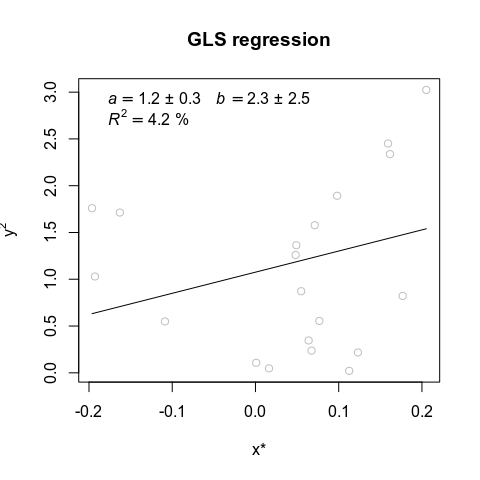Alternatively, we can plot the regression with $$|y|=\sqrt{y^2}$$ on the y-axis to visualize how the standard deviation of $$y$$ is changing with $$x$$. This is the default of the plot function.

plot(gls_mod, ylab = "|y|", xlab = "x*") # with the default: scale = "SD"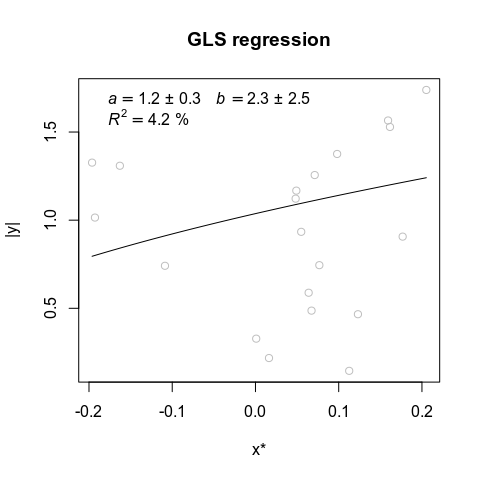Note that the predictor $$x^*$$ in both plots is a transformed $$x$$. Each species $$x^*$$ is a weighted sum of the complete (mean centered) $$x$$-vector. This is because the variance of $$y$$ depends on the historical values of $$x$$ and not its extant value. $$x^*$$ is calculated by the second term in equation 3 in Hansen et al. (2021). The relationship between the original $$x$$ and $$x^*$$ can be plotted by:

plot(gls_mod$data$x_original, gls_mod$data$x, xlab = "x", ylab = "x*")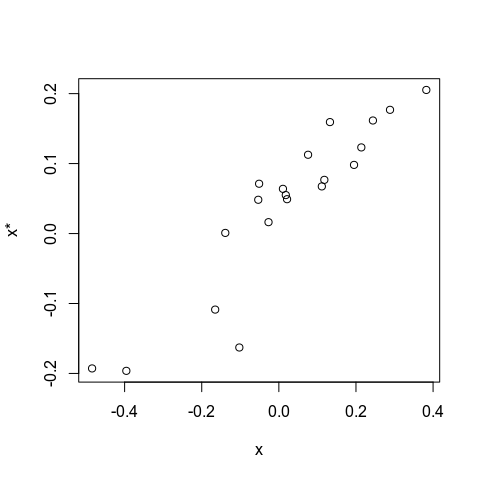# Predictor as geometric Brownian motion

The second model is a model where the predictor $$x$$ evolves according to a geometric Brownian motion (gBM) process, which is equivalent to Brownian motions of the natural logarithm of $$x$$, while $$y$$ is following a Brownian motion with a variance that is linear in $$x$$. This is referred to as model 2 in Hansen et al. (2021). The evolutionary model is

$dy = \sqrt{a + bx} dW_1$

$dx = \frac{1}{2} \sigma^2 xdt + \sigma xdW_2$ where $$a$$ is the evolutionary rate of $$y$$ at $$x=0$$, $$b$$ captures the influence of $$x$$ on the evolutionary rate, and the $$dW_i$$ are two uncorrelated white noise processes. This model will break down when $$a + bx$$ becomes negative and should be seen as an approximation that may be valid for a given range of $$x$$ values as spanned by the species to be used in the analysis. However, in contrast to the above model $$x$$ takes positive values only, which limits the problem of negative $$a + bx$$.

We can simulate data according to the process using:

set.seed(703)
sim_data <- simulate_rate(tree, startv_x=1, sigma_x=1, a=1, b=1, model = "predictor_gBM")

In contrast to the above model, the starting value (root value) of $$x$$ is set to $$1$$ ($$x$$ can only take positive values in this model). In this simulation, the evolutionary rate of $$y$$ at the root is $$\sqrt{a + bx} = \sqrt{1 + 1} = 1.41$$ while the evolutionary rates of the natural logarithm of x, $$\sigma$$ is given by sigma_x = 1.

We can plot the evolutionary dynamics by:

par(mfrow = c(1,3))
plot(sim_data) # defaults to response = "rate_y"
plot(sim_data, response = "y")
plot(sim_data, response = "x")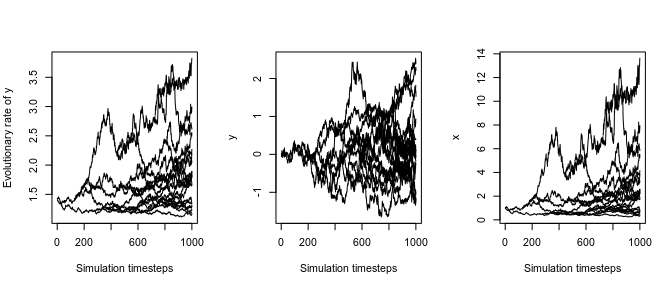The values at the tips:

d <- sim_data$tips head(d) #> species x y #> 1 t7 0.3856851 0.1291090 #> 2 t6 2.1978923 2.4047192 #> 3 t11 0.8027612 2.2119645 #> 4 t1 2.5660087 1.5289728 #> 5 t5 3.2903016 -0.1773951 #> 6 t3 4.1421411 0.9335398 Fitting the iterative GLS model to these data: gls_mod <- rate_gls(x=d$x, y=d$y, species=d$species, tree,
model = "predictor_gBM", silent = TRUE)
round(gls_mod$param, 3) #> Estimate SE #> a 1.206 1.428 #> b 0.188 0.774 #> sigma(x)^2 1.493 0.450 As in Model 1, this output gives the GLS estimates of the parameters of the evolutionary model, where sigma(x)^2 means $$\sigma^2$$ (which equals the squared rate parameter for the Brownian motion process of ln $$x$$) Parametric bootstrapping to get 95% confidence intervals based on the complete process: bootout <- rate_gls_boot(gls_mod, n = 5, silent = TRUE) round(bootout$summary, 3)
#>            Estimate    SE boot_mean boot_median boot_SD   2.5% 97.5%
#> a             1.206 1.428     1.819       1.729   1.265  0.191 3.216
#> b             0.188 0.774    -0.060      -0.225   0.560 -0.621 0.664
#> sigma(x)^2    1.493 0.450     1.631       1.486   0.417  1.285 2.268
#> Rsquared      0.003    NA     0.034       0.028   0.035  0.004 0.084

A plot of the generalized least squares regression fit with $$y^2$$ on the y-axis (i.e. on the variance scale):

plot(gls_mod, scale = "VAR", ylab = expression(y^2), xlab = "x*")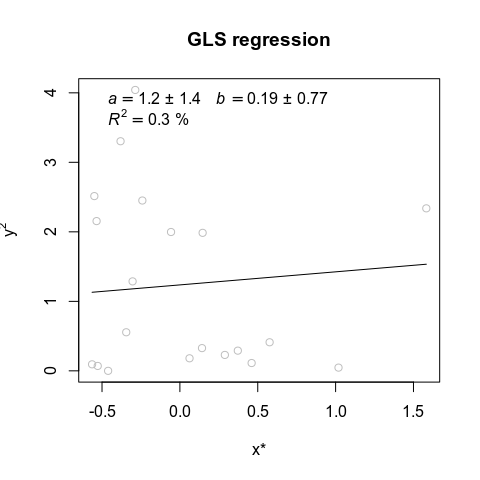As with Model 1 the regression line in the plot does not have $$a$$ as intercept. Instead, the intercept used in the plot is given by the first term of equation 7 in Hansen et al. 2021. The intercept of the regression is the variance in $$y$$ at the theoretical clade mean (i.e. at x* = 0), where the variance of $$y$$ includes components due to estimation error. The slope of the regression is given by the parameter $$b$$ as the explanatory variable plotted is a x-variable transformed by the design matrix.

Alternatively with $$|y|$$ on the y-axis (i.e. on the standard-deviation scale):

plot(gls_mod, ylab = "|y|", xlab = "x*") # with the default: scale = "SD"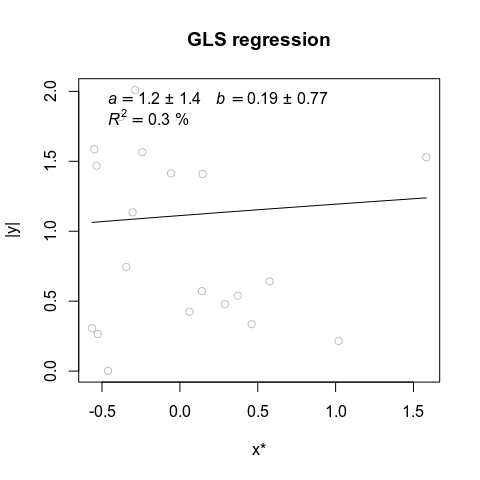And a plot of the transformed x-values ($$x^*$$) on the x-values at the tips. This is because the variance of $$y$$ depends on the historical values of $$x$$ and not its extant value (the transformed x-values are calculated by first standardizing on the root value and centering on the theoretical clade mean before employing the second term in equation 7 in Hansen et al. 2021):

plot(gls_mod$data$x_original, gls_mod$data$x, xlab = "x", ylab = "x*")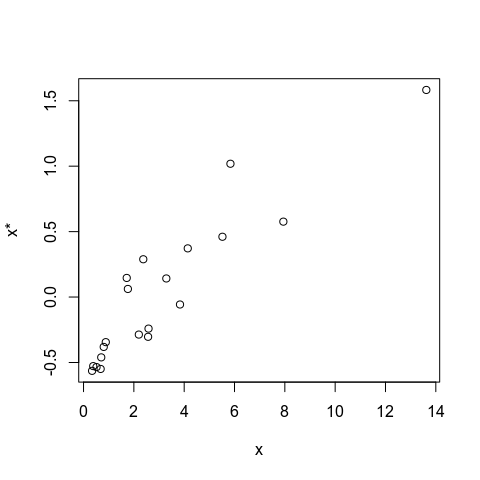# Predictor affects rates of recent evolution

This model stands out from the two above in that we focus on the recent evolutionary process. The species mean trait vector is modeled as $$\mathbf{y} = \mathbf{y}_{macro} + \mathbf{y}_{micro}$$, where $$\mathbf{y}_{macro}$$ is the result of a Brownian motion process and $$\mathbf{y}_{micro}$$ is a micro-evolutionary deviation. The variance of $$\mathbf{y}_{micro}$$ may depend on the predictor variable: $\mathbf{y}_{micro} \sim N(\mathbf{0}, a\mathbf{I} + diag(b\mathbf{x}))$ where $$a$$ and $$b$$ are parameter, $$\mathbf{I}$$ is the identity matrix and $$\mathbf{x}$$ is a vector of species-specific predictor variables. The $$diag$$-function applied to a vector yields a diagonal matrix with the vector along the diagonal. The model assumes that only the recent value of the predictor matters, hence it is treated as a fixed effect in the regression. This is referred to as model 3 in Hansen et al. (2021).

Using the function simulate_rate with model = "recent_evol" we can simulate data with different parameter values.

set.seed(708)
sim_data <- simulate_rate(tree, startv_x=0, sigma_x=0.25, a=1, b=1, sigma_y = 1, model = "recent_evol")

Here, sigma_x is the sample standard deviation of $$x$$ and sigma_y is the BM rate parameter of $$\mathbf{y}_{macro}$$. In this model, we do not simulate data along the phylogeny, so there is no such dynamics to plot. The values at the tips:

d <- sim_data$tips head(d) #> species x y #> t7 t7 -0.09089846 -1.8340332 #> t6 t6 0.20001109 1.8190297 #> t11 t11 0.06363154 -1.5336212 #> t1 t1 0.27625848 -0.3310925 #> t5 t5 0.05598725 0.1462806 #> t3 t3 0.07609817 -3.1574692 We can now fit the GLS model to these data. Note that the model centers $$x$$ and $$y$$ on their respective phylogenetically weighted means. Note also that the useLFO argument can be set to FALSE to speed up the algorithm (particularly useful when bootstrapping). useLFO is an acronym for use “Leave Focal Out”, and controls whether or not the focal species is left out when calculating the species’ mean in the algorithm. The correct way is to use TRUE, but in practice it should have little effect. gls_mod <- rate_gls(x=d$x, y=d$y, species=d$species,
tree, model = "recent_evol", useLFO = TRUE, silent = TRUE)
round(gls_mod$param, 3) #> Estimate SE #> a 0.809 0.528 #> b -2.718 2.588 #> sigma(x)^2 0.053 0.016 #> sigma(y)^2 1.383 NA Again, a and b are the parameters of the evolutionary model, but sigma(x)^2 is the sample variance of $$x$$, and sigma(y)^2 is the squared BM-rate parameter of $$\mathbf{y}_{macro}$$. The standard errors of the estimates are approximate. Parametric bootstrapping can be used to get 95% confidence intervals taking into account the error of the entire process. bootout <- rate_gls_boot(gls_mod, n = 5, useLFO = FALSE, silent = TRUE) round(bootout$summary, 3)
#>            Estimate    SE boot_mean boot_median boot_SD   2.5% 97.5%
#> a             0.809 0.528     0.557       0.367   0.667  0.136 1.597
#> b            -2.718 2.588    -0.050      -1.217   2.263 -1.936 3.080
#> sigma(x)^2    0.053 0.016     0.042       0.042   0.000  0.042 0.042
#> sigma(y)^2    1.383    NA     1.203       1.620   0.828  0.069 1.882
#> Rsquared      0.058    NA     0.111       0.055   0.101  0.041 0.261

Note that $$x$$ is fixed in the bootstrap simulations, and therefore sigma(x)^2 is constant across all bootstrap replicates.

Plotting the the generalized least squares regression with $$y_{micro}^2$$ on the y-axis (i.e. on the variance scale).

plot(gls_mod, scale = "VAR", ylab = expression(y^2), xlab = "x*")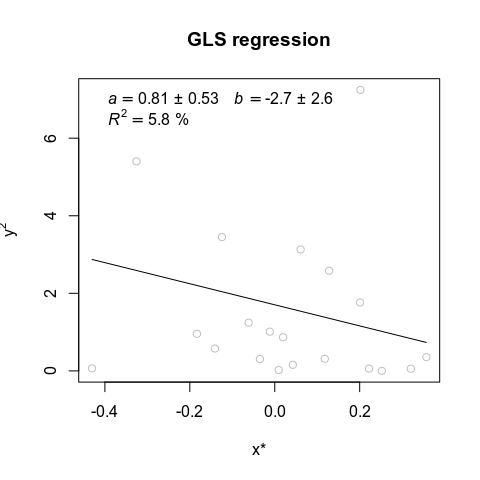The intercept of the regression line shown in the plot is not $$a$$, but the average of all elements in the intercept vector A given by equation 14 in Hansen et al. 2021). The slope is given by $$b$$ as the explanatory variable in the plot are transformed according to the design matrix.

As an alternative to the plot above, we can plot the regression with with $$|y_{micro}|$$ on the y-axis (i.e. on the standard deviation scale). This is the default of the plot function.

plot(gls_mod, ylab = "|y|", xlab = "x*") # with the default scale == "SD"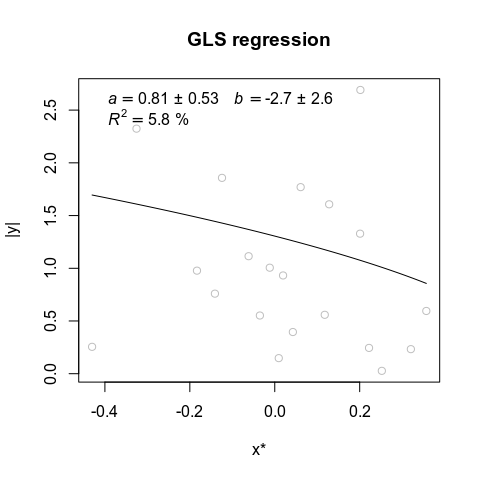Plot of the transformed x-values ($$x^*$$) on the x-values at the tips (the transformed x-values are calculated from the second term in equation 14 in Hansen et al. 2021):

plot(gls_mod$data$x_original, gls_mod$data$x, xlab = "x", ylab = "x*")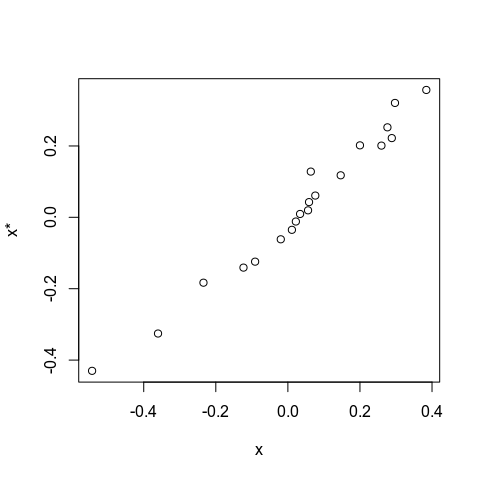Given this model it is also possible to calculate the macroevolutionary predictions of y using the function macro_pred, which is based on equation 10 in Hansen et al. (2021):

# First, extract the relevant parameters:
a <- gls_mod$param["a",1] b <- gls_mod$param["b",1]
sigma2_y <- gls_mod$param["sigma(y)^2",1] # Second, compute microevolutionary variance matrix: V_micro <- a*diag(nrow(d)) + diag(b*d$x)
diag(V_micro)[diag(V_micro) < 0] <- 0  # (Negative variances are replaced by zero)

# Third, compute macroevolutionary variance matrix:
V_macro <- ape::vcv(tree)*sigma2_y

# Last, use macro_pred() to calculate the predictions:
y_macro_pred <- macro_pred(d$y, V=V_macro+V_micro) plot(d$y, y_macro_pred, xlab = "y", ylab = "Macro-evolutionary predictions of y")